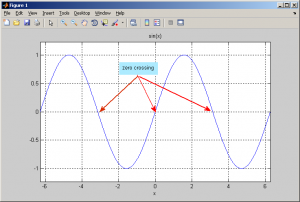# C/C++ Zero Crossing code

Zero-crossing is a commonly used term in electronics, mathematics, and image processing. In mathematical terms, a “zero-crossing” is a point where the sign of a function changes (e.g. from positive to negative), represented by a crossing of the axis (zero value) in the graph of the function. This is picture describe zero crossing location :I have some of data and want to get zero crossing from that data. So, I try to create C/C++ zero crossing code to solve that problem. This my simple C/C++ zero crossing code.

Output from this C/C++ zero crossing function is array with value 0 and 1. Value 1 is location of zero crossing data.

Source :
http://en.wikipedia.org/wiki/Zero_crossing